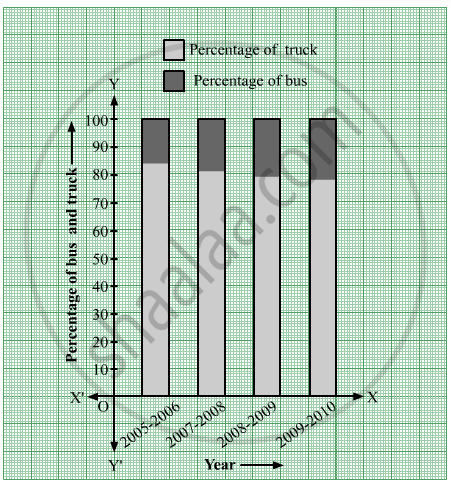Advertisement Remove all ads

# The Following Table Shows the Number of Buses and Trucks in Nearest Lakh Units. Draw Percentage Bar-diagram. (Approximate the Percentages to the Nearest Integer) - Algebra

Graph

The following table shows the number of Buses and Trucks in nearest lakh units. Draw percentage bar-diagram. (Approximate the percentages to the nearest integer)

 Year No. of Trucks No. of Buses 2005-2006 47 9 2007-2008 56 13 2008-2009 60 16 2009-2010 63 18
Advertisement Remove all ads

#### Solution

The following table shows the number of Buses and Trucks in nearest lakh units.

 Year No  of trucks Percentage of trucks No of buses Percentage of buses 2005-2006 47 =47 / (47+9)×100=4700 / 56=83.92≈84 9 =9 / (47+9)×100=900 / 56=16.07≈16 2007-2008 56 =56 / (56+13)×100=5600 / 69=81.15≈81 13 =13 / (56+13)×100=1300 / 69=18.84≈19 2008-2009 60 =60 / (60+16)×100=6000 / 76=78.94≈79 16 =16 / (60+16)×100=1600 / 76=21.05≈21 2009-2010 63 =63 / (63+18)×100=6300 / 81=77.77≈78 18 =18 / (63+18)×100=1800 / 81=22.22≈22

The percentage bar-diagram of the above table is as follows:Is there an error in this question or solution?
Advertisement Remove all ads
Advertisement Remove all ads
Share
Notifications

View all notifications

Forgot password?